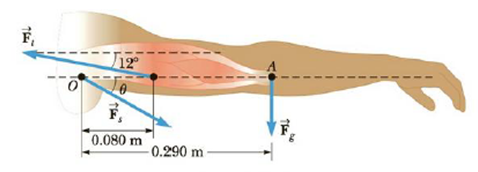Chapter 8, Problem 17P

Chapter
Section
Textbook Problem

Torque and the Two Conditions for Equilibrium17. The arm in Figure P8.17 weighs 41.5 N. The force of gravity acting on the arm acts through point A. Determine the magnitudes of the tension force F → t , in the deltoid muscle and the force F → s exerted by the shoulder on the humerus (upper-arm bone) to hold the arm in the position shown.Figure P8.17

To determine
The magnitude of tension force in the deltoid muscle and the force exerted by the shoulder on the humerus.

Explanation

Given info: The weighs of the arm is 41.5N and the angle of the tension force below the horizontal line is 12° , angle of the tension force below the horizontal line is 12° and the tension force is 724N .

Explanation: The sum of torque about the shoulder joint O is τ=0 and from which, the tension force in the deltoid muscle is determined as follows d1(Ftsinθ)d2Fg=0 . The horizontal component of the force Fs is calculate such that Fx=0=(Fs)xFtcosθ and this expression is rearranged for (Fs)x . The vertical component of the force Fs is calculated such that Fy=0=(Fs)y+FtsinθFg and this expression is rearranged for (Fs)y .

The formula for the magnitude of the tension force in the deltoid muscle is,

Ft=d2Fgd1sinθ

• Fg is the force due to gravity of earth or weight of the arm.
• d1 and d2 are the distances of forces from the point O .
• θ is the angle of tension force below the horizontal line.

Substitute 41.5N for Fg , 0.080m for d1 , 0.290m for d2 , and 12° for θ to find Ft .

Ft=(0.290m)(41.5N)(0.080m)(sin12°)=724N

Thus, the magnitude of tension force in the deltoid muscle is 724N .

The formula for the horizontal component of the exerted by the shoulder is,

(Fs)x=Ftcosθ

• Ft is the tension force

Still sussing out bartleby?

Check out a sample textbook solution.

See a sample solution

The Solution to Your Study Problems

Bartleby provides explanations to thousands of textbook problems written by our experts, many with advanced degrees!

Get Started